## Calculating the residual Signal

In my last post, I started to analyze Dip d792 in detail. But my work was suspended due to a very exciting new dip that has been observed in May 2017.
The first part is here A deep dive into Dip 792 part I.

### Mathematical description

The remaining signal might be the result of a fog cloud, that is in an orbit around the star.

The intensity of this cloud could be calculated if we subtract from the measured signal from Kepler the basic dip. If we choose the timing of the symmetric axis a little bit shifted, to take into account the cloud in the area of the peak of the dip, we find two very smooth exponential decays, as shown in the next graph:The remaining signal shows two exponential growth factors. (missing data points are marked with black dashed circle lines)

Let's look into the details of the plot:
All data are relative to the central peak in a convenient time scale t = t_k-792.740 d (Zero is at the peak of dip 792)

• The raw dip data from Kepler are marked as black vertical x marks.
• The assumed basic dip is presented as a black line and is the mirror of the right half of the dip, not shown in this plot, both data rows use the left linear scaling.
• The remaining density is shown in a logarithmic scaling as shown at the right axis. The first part with very noisy data is presented as grey dots and not further processed.
• The middle part is presented by violent squares and can be approximated by an exponential function with a starting value of 0.022 and a growth factor of tau1 = 0.5298 per day.

This part changes obviously the direction at t = -0.633 d to a steeper growth with a growth factor of tau2 = 1.6655 per day, presented as green x marks.

Both approximations have a very high coefficient of determination, R² = 0.9809 for the first part, which has more noise and R² = 0.9953 for the second steep part.

Here comes a high zoomed plot of the second steep part: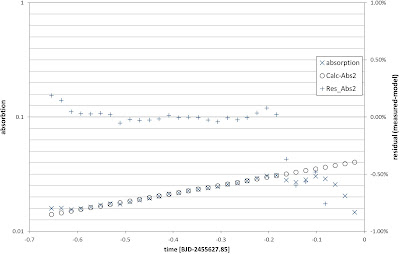The measured signal x marks and the calculated function as circles match in the time range -0.6d to 0.2 day within  0.1% as shown as vertical x marks in the center.
The second plot is focused on the steep function (green in the first plot) in the second part, the deviation is below 0.1% over 0.5 days.

It should be mentioned, that the ratio between the growth factors is 3.143... for whatever reason. (I think it is by pure chance because any intelligent species would use 2 * PI = 6.28... to signal their math level).

But beside this strange coincidence, the exponential decay could have some physical reason. It might be, those dust particles stick together, brightening the "dust" in the orbit.

The two different growth factors may result from two different processes with different efficiency.

Has anyone seen something like this in a comet tail?

Thank you for your attention and give me feedback if you have any comments on this.

## What changed due to 2017 May Dip?

The most exciting thing about the May Dip is, that we now know for sure, the dips at KIC 8462852 are not a Kepler measurement artifact, the Dips are real.
This helps a lot because there is nothing as frustrating as a stupid measurement. I remember the neutrino experiment in Italy, where the speed of light as defined in Einstein's theory was in doubt, only to learn a few weeks later, that a cable was not connected as proposed.

### Time and timing

The next big thing is, that the timing of the dip was not unexpected. The main dips of Kepler happened at day 792 (2011/03/05) and in a time window between day 1518 and 1570, with a strange symmetric peak at day 1539. If the time between this events is in some sense the same, and I try hard not to be too specific than we can propose a period somewhere in the range of 750 to 770 days. Assuming, that we missed a dipping event in 2015 due to the late detection of the strange star by citizen scientists, the next events should take place in the first half year of 2017Dips during the second big dip period between d 1518 and d1570 

But the new dip seems not to be a deep dip beyond 10% flux reduction. The measurement suggests only a <3% dip.The dip has about 98% at peak dipping. (Source: Jason Wright, Twitter)
It should be noted, there have been many dips during the four year period of the Kepler mission with dips of less than 1%, at Tabby's Star, for details see "Meditation over Tabby's Star".

The second interesting thing is the length of the new dip, it seems to last about seven days when we assume the soft start at 16 May 2017 and the sharp end at 19 May 2017 as shown in the graph above. This timing matches the mysterious dip of day 792  very well, a dip that lasted also seven days! It is hard not to believe that this is not a coincidence.Superposition of dip d792 and the May 2017 dip. Be aware of the scaling and sorry for the poor design.
As long as we don't register a stronger peak we can hardly say anything about the internal shape. It may be, that the combination of all available results from multiple sources reduces the error bars significantly.

The spectrometric results are not public at the moment, the only information I could gather is, that there is no significant absorption line and infrared might show a lower absorption as visible light.

### Which Hypothesis gets stronger, which one loose?

There is a very long list of hypotheses  what is going on at KIC 08462852. Let me give my judgement, what has changed at the state of our knowledge today (22 May 2017):

• An object in our local solar system: This seems not to be the case, given the periodicity of 2 years and the knowledge of our solar system. The hypothesis is gone!
• A cloud in the interstellar medium (ISM) in between us and the star. Due to repeated measurement of the signal very low possibility.  Hypothesis not worth to mention anymore!
• Black hole, Hypothesis is gone!
• Comets: This is a tricky one, there are no new supports to a comet, but another observation should here be mentioned: Besides the new dip event, it seems, that the star is still under a global dimming regime as observed by large telescopes. This could hardly be explained by comets. Hypothesis very low chance!
• Planet collision: The big question is, where is the infrared signal! Hypothesis very low chance!
• Star is changing its behavior: We have no model, how a star of that size and age could do that, in addition, the new dip does not support this hypothesis. The hypothesis is gone!
Remaining:
• Something we have not thought about
• Very special thin dust cloud (ring structure) interacting with a magnetic field
• Large-scale Dyson structure of unknown shape (not very good matching the data)
• Star lifting by natural or artificial cause (My favorite)
Star Lifting: Eduard Heindl, A physically inspired model of Dip d792 and d1519 of the Kepler light curve seen at KIC8462852, 2016, https://arxiv.org/abs/1611.08368

I am very happy if you comment on my ideas.

References
 Gary D. Sacco, https://www.reddit.com/r/KIC8462852/comments/56kdfw/95_day_abnormal_equilibrium_of_periodicity_and/
 Jason T. Wright, Families of Plausible Solutions to the Puzzle of Boyajian's Star

 Eduard Heindl, Blogpost 2017, Dip 792 http://some-science.blogspot.de/2017/05/dip-792-at-boyajian-star-kic-8462852.html

## A deep dive into Dip 792 (part I)

The dip 792 of the most mysterious star in the galaxy has a very regular shape and is different from all other signals seen in the Kepler mission. (If new, find an introduction at Dips in Tabby's Star)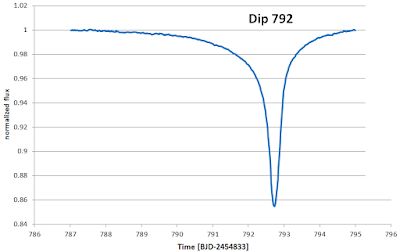The famous Dip of Day 792 at Boyajian Star (Data-Source: NASA Kepler Mission)

### What makes the Dip so unique?

The first thing is, this dip is very deep, about 15% of the light of the star is invisible to the telescope during the peak of the dip. This is a very high number, when we compare that to a transit of a Jupiter like planet, which would absorb about 1% of the starlight and when we remember, that Jupiter is near to the maximum size of a planet.

The second thing is the shape, it is definitely not the shape of a planet, as has been shown by Ksanfomality , The asymmetric structure does not match with a high eccentric planet due to timing and absorption. The same is true for comets, the density of a comet and the homogeneity of the dip is not something we expect to see by well-known comets analyzed in our solar system.

The third mystery is the obvious smoothness of the dip, this is only possible if a relatively simple shape or process is the cause of the dip. we should be very happy to have this dip 792 near the other much more complex and hard to understand dips later in the Kepler mission survey. If we understand D792, we might be able to take the hints to comprehend the other dips.

The fourth mystery is the total time of the dip, that is about 8 days. No massive object, even another star, would occlude this star for so long.

### Analysing the shape

In this post, I do not try to give a possible explanation of the shape as done before , but I look into the characteristics of the shape.

### End and Start of the Dip

The first tricky thing is, to determine the length of the event. The end of the event seems to be easy to determine using a graphical extrapolation. If we look in a high-resolution plot of the data we find, that the dip ends at day 794.85. The uncertainty is within an hour or a few measurement points (0.5h).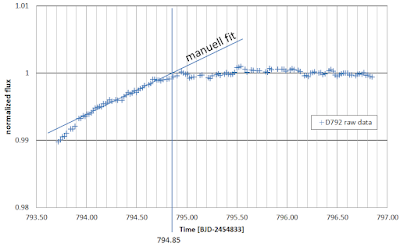Dip 792 ends day 794,85 within a well-defined uncertainty of a few measurement points.

The begin of the dip is much harder to define, due to the very smooth beginning of the dropping light intensity. It seems so, that the density of the absorbing object is fading away with no sharp border as seen in the high-resolution image, be aware of the slightly wider timescale.Dip 792 starts with a very smooth slope of the signal at day 787.

We might get a better understanding of this shape when we assume an exponential decay as shown later in this post.

### Missing Datapoints

A look into the measured brightness at the recovering brightness at Day 792 shows a lot of missing data points. This is more an instrumental issue, but it should be mentioned. For further calculations, the missing data points were substituted by calculated values, using a linear interpolation of the value of the points before and after the gap.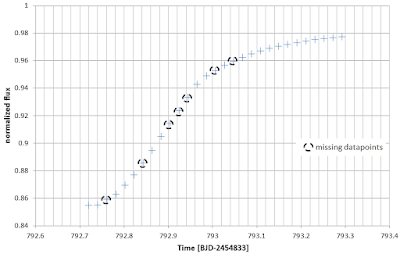Missing data points were calculated by linear interpolation using the two data points at the border of the gap.
The steepness of the curve at the most dramatic part is 0.5%, that compares to a Jupiter sized object, that is leaving the solar disc within one hour.

### Symmetric Dip?

The shape of the dip is not symmetric, but what, if we assume, there are two parts, one symmetric event (basic dip) and another part (fading part) which is adding to the symmetric part and resulting in the visible dip?

This calculation can be done if we assume, that the first part of the basic dip has the same shape as the second part. The second part is well known and the only thing is, to generate a mirror picture with a meaningful axis. To generate an easy to read mirror image, the missing data points during the measurement of Kepler were substituted by a linear interpolation of the available border points of the gap, in most cases, only one point was missing, resulting in a minor error.

The result is visible in the next plot, where both plots are visible. Blue is the original time series, red is the same graph, mirrored at the axis at the time point 792.73d.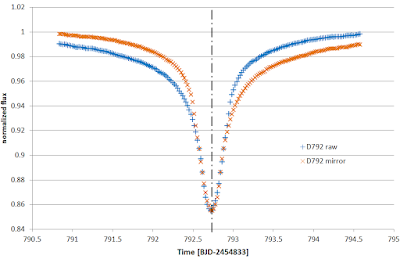The symmetric assumption of the dip 792.

We can now synthesize the basic dip and the fading part. First look at the basic dip:The basic dip is an artificially constructed dip using symmetric assumptions.
The basic dip has now a defined start and end, using the point of symmetry, 792.73d, and the value found for the end of the dip at 794.85d. We define this information:
Start of basic dip: t1 = 790.61d
Maximum: ts = 792.73d
End of basic dip: t2 = 794.85d
Duration: T =  4,24d

Further analysis of this shape might be interesting, but is not part of this post, maybe I will discuss this in another post.

### The remaining Signal

Very interesting is now the question, what happens to the remaining signal. The remaining signal, calculated by subtracting the basic dip from the measured value, is shown in the next plot:The remaining fading part, if we subtract the artificial basic dip from the measured data.
The fading part seems to have an exponential character. This should be analyzed further.
The best way to see the exponential character is, to plot the data in a logarithmic scaling in the absorption axis.

To optimize the result, the data before day 791 are reduced in noise, by not subtracting the symmetric signal but by using a constant baseline with the value one. Otherwise, meaningless noise and fluctuations from the baseline beyond the basic dip would appear.Log-plot of the absorption in the remaining signal after subtraction of basic dip. Different colors mark different slopes.
The log-plot shows at least four different areas. At the left part, there is a lot of noise and a relatively steep slope. The blue squares mark an area with a constant slope, the exponential factor is 0.46 1/day. Around day 92 the slope increases significantly to a higher value of 1.32 1/day. The last points at day 792.55 and following, don't show any pattern and may result from a remaining error concerning the unknown exact shape of the basic dip.

Here comes the second part.

2017 May 19: We have a new dip, I publish this, and wait for new results.

Live: https://youtu.be/eYpIGZS8nJc

## When will the next Dip appear at Boyajians Star?

In the last posts (d792, d1519), I described a model of the different large dips. This model is based on a beam that lifts matter from the star surface into an orbit. Due to the geometric situation, it should be possible to calculate the rotation speed of the beam. Actual the rotation speed is part of the parameter set in the shape calculation of the beam.
In this post, I will suggest a possible period.

### The parameter rotation speed

The timing of the beam calculation is based on the equation

α(t) = ((BJD-2454833) – t) ω

BJD-2454833 is the time for all the Kepler data , based on the BJD time,
t0 describes the dip at day 792 and has the exact value 792.7216d
ω is the rotation speed and has to be fitted to the data.
If we know the exact value, we caught the rotation period of the beam and can calculate the reappearance of more dips.

In the first paper, I suggest ω has the value of 1.00E-02[1/d]. This correlates to a period of circulation of 628 days (2pi*100). The loosely guess of the 100 was done with no special precision.
In a review of the situation, I changed the value from 100 to 115,67 (Distance from d792 to 1519) and checked the effect to the shape of the calculated dips, with a special concentration on the lower part of the absorption. As shown in figure 1.Figure 1: Dip 792 optimized right part
This fits very nicely in the in the area where the error was considered, shown as red error bars. A calculation when I change the period by one-day results already in a higher error in this region.
The other part of the plot is not that perfect, but other factors are not optimally implemented in the model, like the beam cross section.
To remember, in the right part under investigation, only the beam generates a shadow, the shape of the beam is not as influential as in the area of the deep dip.

### Dips in the context

If we use the flux calculation for the whole Kepler period, we can see the repetition of the calculated flux as shown in figure 2:

Figure 2: Flux over the whole period (Right scale "Calculated Flux" lowered for good readability)

We see of course the tick at d792, which is the base of calculation. But we see also a dip at day 429, and day 1156, both are in some way an artifact, because the equation works with the absolute sin and contains in this sense a beam that would be on the other side of the star.
But there is an interesting detail, very near to this dips, we see in each case a small dip in the measure of Kepler. Let's have a look at the detail of dip d429 in figure 3:

Figure 3: Timing of d426 and the timing of the dip artifact.

It might be, that the technology behind star lifting generates some small beam at the opposite side of the star, this might be due to a magnetic field. But this can also be a pure coincidence. Due to the fact, that at day 1150 another similar dip is visible, should focus some resources to understand this coincidence.

### The second appearance

At day 1519, we see the second appearance of our dip d792. The shape has changed but an interesting detail is still there, look into figure 4:Figure 4: The dips around day 1519

In this plot, the shape of the d792 reappears due to the period of 726.78 days. Other elements add to that dip as discussed in the post 1519, but there is one amazing element, at day 1518, the flux recovers just to the level of the remaining effect of d792, but not more. This might be interpreted in a way, that this is, in fact, the separate structure of dip 792 appearing together with other elements of beams which reduce the flux at other times in the interval.

### When will the next Dip appear

The most interesting question is, when will the next Dip appear?
I make the prediction as following in a table:

Time of dips in UTC time, Kepler starts at t = 0 (BJD-2454833): 2009-Jan-1 11:29:59, I used the converter from Ohio State University

dip      BJD              UTC
-   2454833      2009-Jan-1  11:29:59 (Start of Kepler time)
792  2455625.722  2011-Mar-5   4:49:40
1519  2456352.500  2013-Mar-1   0:35:59
2246  2457079.278  2015-Feb-25 18: 4:19
2973  2457806.057  2017-Feb-21 13:58: 4
3700  2458532.835  2019-Feb-18  7:26:24
4427  2459259.614  2021-Feb-14  3:20: 9

(I am not completely sure about the time conversion, if someone is here an expert, he may check the Kepler start time) I have now revisited the timing conversion, using Eastman, Jason tool  and U.S. Naval Observatory Astronomical Applications Department the result is slightly different but very well within the error margin. Tuesday, A.D. 2017 Feb 21, 13:18:25.1 (JD 2457806.054457)

On February 21st should see a dip!
I am very curious to see if the result matches the prediction.

I am very curious to see the result.

Reference:

 Eastman, JasonSiverd, RobertGaudi, B. Scott,
 Achieving Better Than 1 Minute Accuracy in the Heliocentric and Barycentric Julian Dates
DOI 10.1086/655938

## Solving the puzzle of Dip 1519

This post continues the analysis of different dips seen by the Kepler telescope at Boyajian's star (KIC 8462852). To understand the discussion, I recommend reading the analysis of Dip 792, because I use the same basic model.

### Again a Starlift model

The very useful stairlift model of the last post is reused to understand the very complex signature of the dips around day 1519, as presented in fig 1.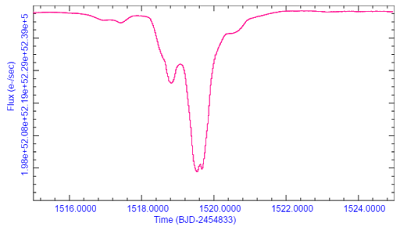Fig 1: Komplex deep Dip 1519
The dip includes a very deep double dip, with 22% absorption, and an asymmetric basic structure, similar to dip 792.
My idea was, to use the simulated shape of a starlift with "smoke" as described in detail in Dip 792, to understand the shape 1519. Therefore the shape was positioned three times in the time frame with a different position in time and different absolute absorption.
The result is shown in fig 2.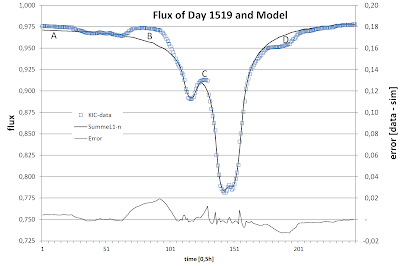Fig 2: A first attempt to reproduce dip 1519

The simulated black line does not reproduce the blue measured values, but some very significant elements are well done. First of all, the double asymmetric main dip fits just perfect. And this was done by simply adding the model of Dip 792 with the same intensity, the factor is in both cases exact 1.0 (one)!
The first deep dip is deeper than the second, the reason is, that the "smoke" of the second dip deepens the first. The numerical distance in time was set to 5h. The length of the starlift beam is 1.50 higher as in dip 792. This also means that the orbit of the smoke in this simulation is by a factor 1.50 further away as in dip 792.

To complete the picture, a third starlift beam was introduced 12.5h before the main dip.
The factor for this dip is 0.36789. Ever seen this number? It is 1/e, but it seems so, that value is by accident similar.
e is the very well known Euler number 2.7182.... the number of natural growth and the mathematical basis for the success of any civilization. (More about at wikipedia)

### Some Problems

But the simple model does not reproduce the measured line in all parts. In area A, B and C, the signal is brighter than it should be. There are two possible explanations:
1. The starlift beam had some interruptions and so the smoke has some breaks.
2. The material of the smoke was used for construction and is no longer at this place in the orbit.
If we like solution 2, then it is not to hard to understand bumper D in fig 2. It might be some material in the orbit, it could even be a mirror to power the starlift itself. But this is pure speculation.
A better solution is given as a hard puzzle to the reader.

Thank you for reading and please give me feedback.
Hopefully, a paper, concerning this research is soon completed.

## Calculation of Dip 792d with Star lifting

This post is the second part of the calculations to understand the shape of the dip at day 792 of Boyajian's star (KIC 8462852). For part one visit post: "Do we see Star lifting".

### The Beam is Bent

The Dip day 792 is not symmetrical, therefore we have to introduce a beam, that is not perfect on a straight line from the center of the star. it is not difficult, to find reasons why the beam of the matter is bent in a direction. A simple reason is in Kepler dynamics during departure from the surface.
As long as we don't understand the process in detail, I will again start with a simple model and try to fit the model with the data.
Figure 1 describes the new model of the beam. The beam starts at the surface of the star, and with height H it starts to have an optical density due to reduced temperature and therefore is no longer ionized.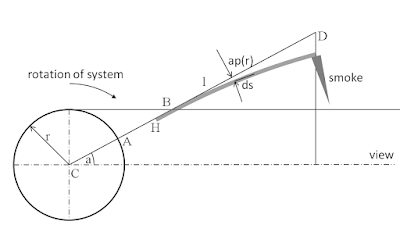Fig 1: Properties of the physical model.

The beam is now modeled with small fractions of the length ds which are distorted against the direction angle depending on the distance l. At the end of the beam, the "smoke" comes to a rest and is distributed in this orbit by an exponential law, the density is maximal at the beam end and decays with distance in the bending direction.
For the simulation, the beam contains 105 elements. Each represents a beam length of 5 star radii, The first 5 star radii (first element in the simulation) are transparent due to the high temperature near the star surface.
Each simulated beam element has a transparency of 0,9984722. Each element is a little bit bent by
ap = -0,3971 day.
If an element is within the line of sight between Kepler and KIC 8462852, the resulting transparency is calculated by the multiplication of the transparency of every element.
At the end of the beam, the material enters an orbit, for some reason, and is accumulated. The optical density of the accumulated material decays by an exponential function d = d0 exp(a*w) thereby d0 = 0,001588, w = 3,30025 [1/day]. (The unit hour is used and could be converted in an angle if the distance and rotation period would be known). The value of the simulation was always averaged over 2.5h to adopt a little bit the shape of the unknown optical elements.
The result of this simple physical simulation is shown in fig 2:Fig 2: Measured and simulated flux using the model
The model, containing only simple and plausible parameters fits within the limits of the Kepler data precision. The value of the free parameters, namely bending, optical density and the exponential function, were optimized manually.

I did not expect a result with such a low error.

There are some aspects not included in the simulation: an exact cross-section of the beam, surface flux of the star (it was assumed to be constant).

### Other posts related to Tabbys Star

Dips part two
A simple model of Dip 792

## The Try of an Explanation of the Dip at day 792

In the last time, there has been the suggestion, that the very strange dip around day 792 might be the signature of star lifting. A reasonable explanation of star lifting can be found at Wikipedia.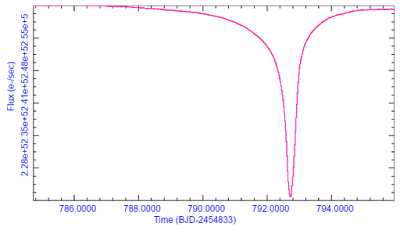Fig 1: The dip at day 792 has a very interesting homogenous shape.

The basic Idea is, that a super civilization is able to harvest matter from the local star by magnetic or other means. This is quite difficult, due to the high temperature at the surface of the star. Therefore a beam of matter, similar to a natural solar solar flare has to be produced. I don't go into the technical details how or if this is possible, but I try to simulate the visible lightcurve of such an activity.Fig 2: Natural solar flare at our Sun.

### The most simple model

(A more complex model is described here "Dip 792 in Detail")
To generate the situation, we start with a very simple model. A long beam from the star points radially away from the center of the star. by accident, we are in the line of sight and see the beam crossing the star.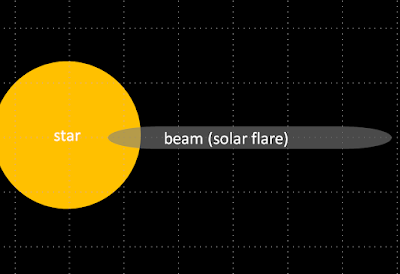Fig 3: Simple model, a beam of matter, pointing away from the star.

To describe this model, we assume, that the beam is rotating around the star and a fraction of the beam absorbs the starlight on our line of sight.
The amount of dimming is then a function depending on geometric factors and the rotation angle as shown in fig 4 viewing the situation from the rotation axis of the system.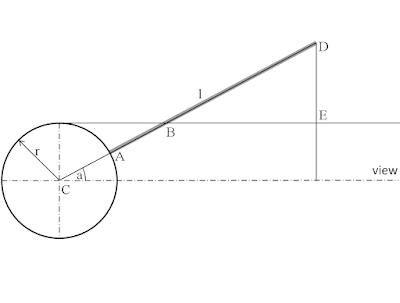Fig 4: Geometric situation, Kepler looks from the right side to the star with center C.

The star has a radius of r and the center is marked by C, a beam with an optical density d, at the surface of the star starts at point A and ends at point D. The dimming is proportional to the length, of the distance |AB|, because only this part of the beam covers our line of sight. We can calculate the distance CB, depending on the angle a, it is

|CB| = r/sine(a)

len(AB) = r/sine(a) - r  (1)

The angle a is depending on the time t, and the angular velocity w, by which the beam rotates around the star like a hand at a clock.
It is convenient to set the time t to zero when a = 0. To suppress the infinite length of AB at a = 0, we have to take into account, that the beam is not infinite, but has the length AD. The equation (1) holds therefore only as long as the beam does not cross the sightline BE. This happens at the angle

ac = arcsine( r / |CD| )

ac = arcsine( r / ( |AD| + r ))  (2)

The measured flux f(t) is then calculated, assuming f0 is the brightness of the star, by

f(t) = f0 - d ( r / sine( w t ) - r)   in the case {||wt|| > ac}

f(t) = f0 - d |AD|   in the case  {||wt|| < ac}   (3)

Let's have a look at our dip at day 792: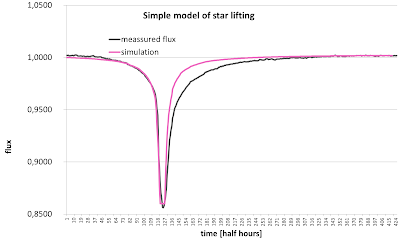Fig 5: Very simple model of star lifting. (Sorry, for some reason this image is flipped in time)
Although we have used the most simple model, the left part of the graph is astonishingly similar to the measured dip. The parameters used for equation (3) are d = 0.16, f0 = 1, r = 1, w = 0,14 [1/30min].

### An inhomogeneous absorbing beam

A further approximation to the real situation can start with the optical density of the beam. At the surface of the star, the beam has the same temperature as the solar surface and will not absorb any light. By leaving the surface the beam cools down and the atoms might absorb light by ionization. Again, I try a very simple model, the temperature is then depending from the visible surface of the sun, depending on the height.
My geometric model is plotted in fig 6.Fig 6: Temperature of the beam as a function of height |AB|

At the point B the beam has a height above the star of |AB|=h. The visible angle a is therefore given by the geometric relationships in the rectangular triangle BCD.

|AB| + r = |BC|
and
sin(a)=r/|BC|

sin(a)=r/(|AB| + r)

a = arcsine(r/(h + r))   (4)

The visible cone P is, therefore, relative to the 2 pi surface situation

P = 2 pi sin(a) /2 pi

P = (r/(h+r))   (5)

A plot of this function is shown in fig 7.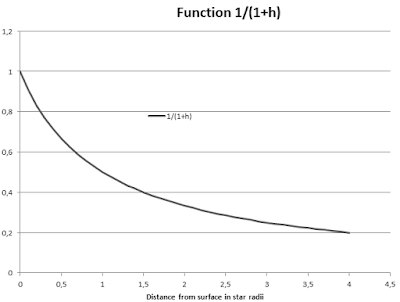Fig 7: Showing the surface of the star, a beam sees at a distance from the star

To keep things simple, we define a height, where the reionization happens. The function in fig 7 drops fast and the black body radiation is depending on the fourth power, so I guess with a height of two-star radii, the beam is reionized.

To include this in our model, we replot a modified figure 4 in fig 8.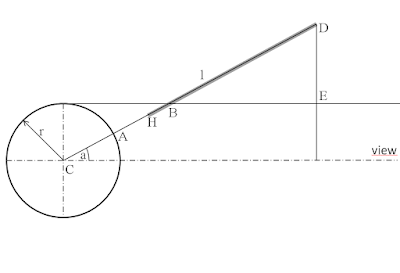Fig 8: Modified beam with optical density starting at H
In the new model, the beam starts to have an optical density, starting at the point H and going up to D. Using this, we can replot the simple calculation from fig 5 again, only presenting the falling edge for better visibility: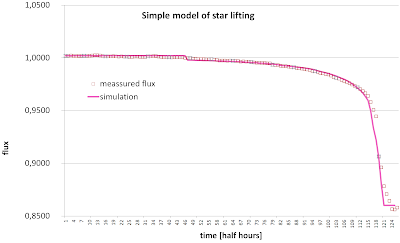Fig 9: The model matches the measured flux better in the first part.

Although the model is still very simple, the match between measured flux and model is now also in the first part good. Be aware, that the ionization doesn't happen instantly and has to be modeled by a more sophisticated model the basic effect of a certain threshold seems to exist.
The steep side of the dip is not perfect, this may be due to the inhomogeneous radiation density of the star, another point that a better model should include.

In the next post, I will try to model the very steep rising edge by bending the beam.

If anyone wants to support me with efficient computer models, he is invited please drop an email heindl(a)gmail.com

### Other posts related to Tabbys Star

Dips part two
A more complex model of Dip 792